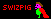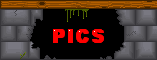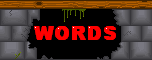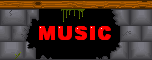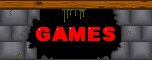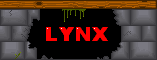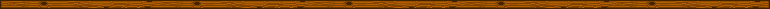Cell (750KB, Windows only, sorry)# How to play

YOU ARE THE RED SQUARE, and you must shoot everything else. More points are scored the more enemies are on screen.

# Initial controls

WASD to move, cursor keys to fire, space to activate your smart bomb.
OR
Joystick, initially set up for (on my Logitech pad) left stick to move, right stick or right-hand diamond buttons to fire, plus button '6' as bomb.

ESC to quit current game, or from title screen to exit the game totally. Any key, or joystick shoot-up, to start.

# Enemies

 RUNNER: Keeps going in one direction SPREADER: Sits there, spawning a new spreader periodically WALL: Slowly travels across the grid, growing with each step HOMER: Constantly moves towards the player BOMB: Shoot it before it explodes to gain a bomb. Otherwise it'll explode into... SHRAPNEL: ...which flies off in all 8 directions

# config.txt

This file configures the control setup. Note that the joystick axis and button controls will start from zero - on my pad, the button marked '1' will actually register as button 0, '2' as 1, etc. It can contain the following:

## Joystick axis control:

use_axis_xmove - the next line contains the number of the joystick axis to use for horizontal movement
use_axis_ymove - the next line contains the number of the joystick axis to use for vertical movement
use_axis_xshoot - the next line contains the number of the joystick axis to use for horizontal shooting
use_axis_yshoot - the next line contains the number of the joystick axis to use for vertical shooting
invert_axis_xmove
invert_axis_ymove
invert_axis_xshoot
invert_axis_yshoot - invert the relevent axis

## Joystick button control:

joy_buttons - followed by 9 lines specifying what button to use for move up, move down, move left, move right, shoot up, shoot down, shoot left, shoot right, and bomb (in that order).

## Keyboard control:

keys - followed by 9 lines of (decimal!) numbers specifying what keys to use, in the same order as joy_buttons above. Here's a big list pinched from the SDL headers:
 ``` SDLK_UNKNOWN = 0, SDLK_FIRST = 0, SDLK_BACKSPACE = 8, SDLK_TAB = 9, SDLK_CLEAR = 12, SDLK_RETURN = 13, SDLK_PAUSE = 19, SDLK_ESCAPE = 27, SDLK_SPACE = 32, SDLK_EXCLAIM = 33, SDLK_QUOTEDBL = 34, SDLK_HASH = 35, SDLK_DOLLAR = 36, SDLK_AMPERSAND = 38, SDLK_QUOTE = 39, SDLK_LEFTPAREN = 40, SDLK_RIGHTPAREN = 41, SDLK_ASTERISK = 42, SDLK_PLUS = 43, SDLK_COMMA = 44, SDLK_MINUS = 45, SDLK_PERIOD = 46, SDLK_SLASH = 47, SDLK_0 = 48, SDLK_1 = 49, SDLK_2 = 50, SDLK_3 = 51, SDLK_4 = 52, SDLK_5 = 53, SDLK_6 = 54, SDLK_7 = 55, SDLK_8 = 56, SDLK_9 = 57, SDLK_COLON = 58, SDLK_SEMICOLON = 59, SDLK_LESS = 60, SDLK_EQUALS = 61, SDLK_GREATER = 62, SDLK_QUESTION = 63, SDLK_AT = 64, /* Skip uppercase letters */ SDLK_LEFTBRACKET = 91, SDLK_BACKSLASH = 92, SDLK_RIGHTBRACKET = 93, SDLK_CARET = 94, SDLK_UNDERSCORE = 95, SDLK_BACKQUOTE = 96, SDLK_a = 97, SDLK_b = 98, SDLK_c = 99, SDLK_d = 100, SDLK_e = 101, SDLK_f = 102, SDLK_g = 103, SDLK_h = 104, SDLK_i = 105, SDLK_j = 106, SDLK_k = 107, SDLK_l = 108, SDLK_m = 109, SDLK_n = 110, SDLK_o = 111, SDLK_p = 112, SDLK_q = 113, SDLK_r = 114, SDLK_s = 115, SDLK_t = 116, SDLK_u = 117, SDLK_v = 118, SDLK_w = 119, SDLK_x = 120, SDLK_y = 121, SDLK_z = 122, SDLK_DELETE = 127, /* End of ASCII mapped keysyms */ /* Numeric keypad */ SDLK_KP0 = 256, SDLK_KP1 = 257, SDLK_KP2 = 258, SDLK_KP3 = 259, SDLK_KP4 = 260, SDLK_KP5 = 261, SDLK_KP6 = 262, SDLK_KP7 = 263, SDLK_KP8 = 264, SDLK_KP9 = 265, SDLK_KP_PERIOD = 266, SDLK_KP_DIVIDE = 267, SDLK_KP_MULTIPLY = 268, SDLK_KP_MINUS = 269, SDLK_KP_PLUS = 270, SDLK_KP_ENTER = 271, SDLK_KP_EQUALS = 272, /* Arrows + Home/End pad */ SDLK_UP = 273, SDLK_DOWN = 274, SDLK_RIGHT = 275, SDLK_LEFT = 276, SDLK_INSERT = 277, SDLK_HOME = 278, SDLK_END = 279, SDLK_PAGEUP = 280, SDLK_PAGEDOWN = 281, /* Function keys */ SDLK_F1 = 282, SDLK_F2 = 283, SDLK_F3 = 284, SDLK_F4 = 285, SDLK_F5 = 286, SDLK_F6 = 287, SDLK_F7 = 288, SDLK_F8 = 289, SDLK_F9 = 290, SDLK_F10 = 291, SDLK_F11 = 292, SDLK_F12 = 293, SDLK_F13 = 294, SDLK_F14 = 295, SDLK_F15 = 296, /* Key state modifier keys */ SDLK_NUMLOCK = 300, SDLK_CAPSLOCK = 301, SDLK_SCROLLOCK = 302, SDLK_RSHIFT = 303, SDLK_LSHIFT = 304, SDLK_RCTRL = 305, SDLK_LCTRL = 306, SDLK_RALT = 307, SDLK_LALT = 308, SDLK_RMETA = 309, SDLK_LMETA = 310, SDLK_LSUPER = 311, /* Left "Windows" key */ SDLK_RSUPER = 312, /* Right "Windows" key */ SDLK_MODE = 313, /* "Alt Gr" key */ SDLK_COMPOSE = 314, /* Multi-key compose key */ /* Miscellaneous function keys */ SDLK_HELP = 315, SDLK_PRINT = 316, SDLK_SYSREQ = 317, SDLK_BREAK = 318, SDLK_MENU = 319, SDLK_POWER = 320, /* Power Macintosh power key */ SDLK_EURO = 321, /* Some european keyboards */ SDLK_UNDO = 322, /* Atari keyboard has Undo */ ```